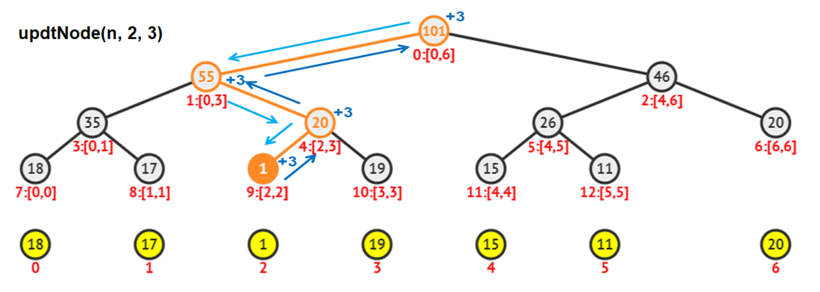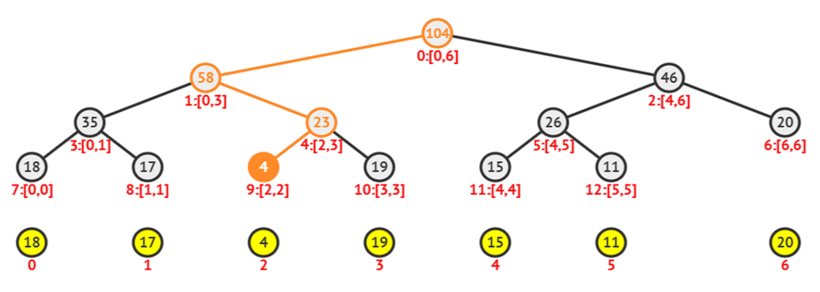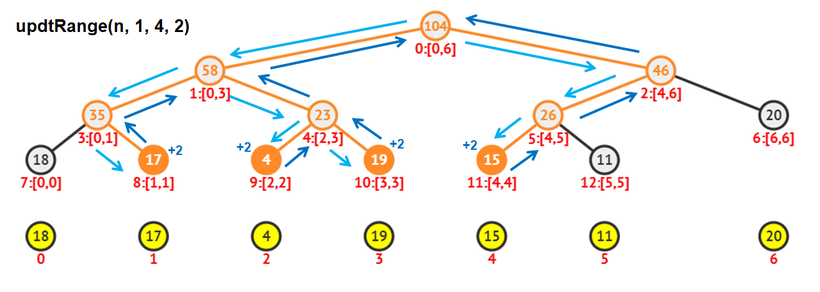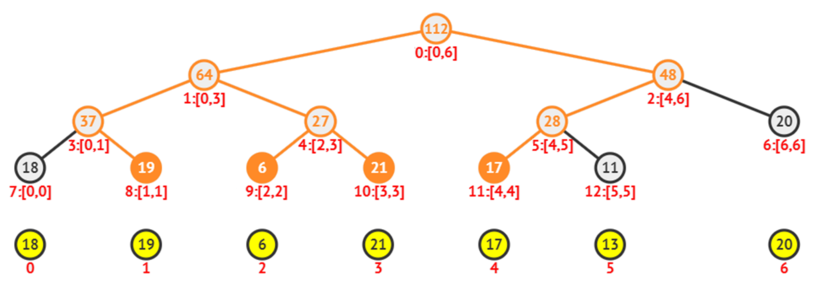# Node and range updates in Segment Tree using C++

In this tute, we will discuss node & range updates in segment tree using C++. To get clear knowledge, read till the end. Before getting into updates, make sure you get the basics of the creation of a Segment tree.

Key points to remember:

• Segment trees are Full Binary trees.
• All elements of the array are at leaves.
• Each intermediate node contains the cumulative value of a range in the array.
• To access a node/range you always have to start from the root and go to the node at the next level whose range contains the idx to be visited.
• Since it is a Full Binary tree, it always has either 2 children or no children.

To visualize how full binary trees get altered upon the addition of a new node, try your inputs here.

In this post, let us choose the Sum Segment tree for the sake of simplicity. Keep in mind that, Segment trees without lazy propagation are useful for Range queries and Point updates.

Let us consider the following array:For node updates, an index idx of the array and value V are given.  We must update all the nodes of the tree which contains the idx in its range.

Algorithm:

1. Firstly, start at the root node which covers the entire array.
2. If it has children, check both left and right nodes and enter into the child which contains the idx.
3. Continue this till the target idx is reached.
4. Because we are using a recursive approach, we would trace back the same route to the root.
5. Finally, add the value V to all the nodes in this route.After update,For range updates, range l & r of the array and value V are given. We must update all the nodes of the tree which contains some elements of ar[l..r] in its range.

Algorithm:

1. Firstly, start at the root node which covers the entire array.
2. Check both left and right nodes and enter the child or both children which contains some elements in their range.
3. Continue this till the target leaves are reached.
4. At the leaves, update the node with +V.
5. While tracing back to the root, at each intermediate node, recalculate that node’s value by simply adding the two child nodes.
`tree[idx] = tree[2*idx+1] + tree[2*idx+2];`After update,Implementation:

Following is the implementation of Sum Segment Tree. The program implements construction, node update, range update on Segment Tree.

```// C++ program to show Segment Tree - node & range updates
#include<bits/stdc++.h>
using namespace std;

#define MAX 100010
int tree[MAX];
vector<int> ar;

/* Function to display elements of the Segment Tree
level by level. */
void showSegTree(int n)
{
int i, j, h = ceil(log2(n));
for(i=0 ; i<=h ; ++i)
{
for(j=0 ; j<pow(2, i) ; ++j)
cout<<tree[int(pow(2, i)-1 + j)]<<' ';
cout<<endl;
}
}

/* Function to construct the Segment Tree recursively.
At each node, if it is not the leaf, two children are
processed further. Otherwise, the leaf is assigned an
array alement. strt and end are range in the array,
idx is the index used in the segment tree */
int constSegTree(int strt, int end, int idx)
{
// Saves tree[idx] = ar[strt] and then returns tree[idx]
if(strt == end)
return tree[idx] = ar[strt];

int mid = (strt + end) / 2;
return tree[idx] = constSegTree(strt, mid, 2*idx+1) +
constSegTree(mid+1, end, 2*idx+2);
}
void constSegTree(int n)
{
constSegTree(0, n-1, 0);
}

/* Function to update a particular node of the array in
Segment Tree. pos is the desired index in the array.
val is the value to be added */
void updtNode(int pos, int val, int l, int r, int idx)
{
// pos not inside the node's range
if(pos < l || r < pos)
return;

// Update all nodes in root <-> pos path
tree[idx] += val;

// Leaf node reached , i.e, No children
if(l == r)	return;		//Exit condition

int mid = (l + r) / 2;
updtNode(pos, val, l, mid, 2*idx+1);
updtNode(pos, val, mid+1, r, 2*idx+2);
}
void updtNode(int n, int pos, int val)
{
updtNode(pos, val, 0, n-1, 0);
}

/* Function to update a range of array elements in the Segment
Tree. strt and end are the range in the array. val is the value
void updtRange(int strt, int end, int val, int l, int r, int idx)
{
// Completly ouside the desired range
if(end < l || r < strt || l>r)
return;

// Leaf node reached
if(l == r)
{
tree[idx] += val;	// Update the leaves
return;
}

int mid = (l + r) / 2;
updtRange(strt, end, val, l, mid, 2*idx+1);
updtRange(strt, end, val, mid+1, r, 2*idx+2);

// Update the intermediate nodes
tree[idx] = tree[2*idx+1] + tree[2*idx+2];
}
void updtRange(int n, int strt, int end, int val)
{
updtRange(strt, end, val, 0, n-1, 0);
}

int main()
{
int n = 7;
ar = {18, 17, 1, 19, 15, 11, 20};

constSegTree(n);

cout<<"n = 7, ar = {18, 17, 1, 19, 15, 11, 20}"<<endl;
showSegTree(n);

updtNode(n, 2, 3);

cout<<"\nupdtNode(n, 2, 3):"<<endl;
showSegTree(n);

updtRange(n, 1, 4, 2);

cout<<"\nupdtRange(n, 1, 4, 2):"<<endl;
showSegTree(n);

return 0;
}```

Output:

```n = 7, ar = {18, 17, 1, 19, 15, 11, 20}
101
55 46
35 20 26 20
18 17 1 19 15 11 0 0

updtNode(n, 2, 3):
104
58 46
35 23 26 20
18 17 4 19 15 11 0 0

updtRange(n, 1, 4, 2):
112
64 48
37 27 28 20
18 19 6 21 17 11 0 0```

Time Complexity:

Tree Construction: O(n)

Node update: O(Log2n)

Range update(k elements): O(k * Log2n)

Bonus: If you still find it difficult to understand about node & range updates in segment trees, remove all comments in the code and start tracing for your inputs.